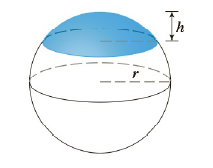Chapter 5.2, Problem 49E

Chapter
Section
Textbook Problem

# Find the volume of the described solid S.A cap of a sphere with radius r and height hTo determine

To find:

The volume of a cap of a sphere with radius r and height h.

Explanation

1) Concept:

Definition of volume:

Let S be a solid that lies between x=a and  x=b. If the cross sectional area of S in the plane Px, through x and perpendicular to the x-axis, is  A(x), where A is a continuous function, then the volume of S is

V=limni=1nAxi*x=abAxdx

2) Given:

Radius of sphere is r and height of cap is h.

3) Calculation:

Consider a cross section of the cap at height h from the origin as shown in above figure.

To find the cross section,

Consider a circle  x2+y2=r2

Subtract y2  from both sides,

x2=(r2-y2)

Taking square root to find the value of x,

x=r2-y2

From the figure, limits of integral are from r-hto r

### Still sussing out bartleby?

Check out a sample textbook solution.

See a sample solution

#### The Solution to Your Study Problems

Bartleby provides explanations to thousands of textbook problems written by our experts, many with advanced degrees!

Get Started

#### A sample of n = 9 scores has X = 108. What is the sample mean?

Essentials of Statistics for The Behavioral Sciences (MindTap Course List)

#### f(x) = 3x3 x2 + 10; limxf(x); and limxf(x) x 1 5 10 100 1000 f(x) x 1 5 10 100 1000 f(x)

Applied Calculus for the Managerial, Life, and Social Sciences: A Brief Approach

#### 2. Define the terms population, sample, parameter and statistic.

Statistics for The Behavioral Sciences (MindTap Course List)

#### For y = x sin x, y = _____. a) x cos x b) x cos x + 1 c) cos x d) x cos x + sin x

Study Guide for Stewart's Single Variable Calculus: Early Transcendentals, 8th